# Random Variables Statistics 16 A random variable assumes

• Slides: 32Random Variables Statistics 16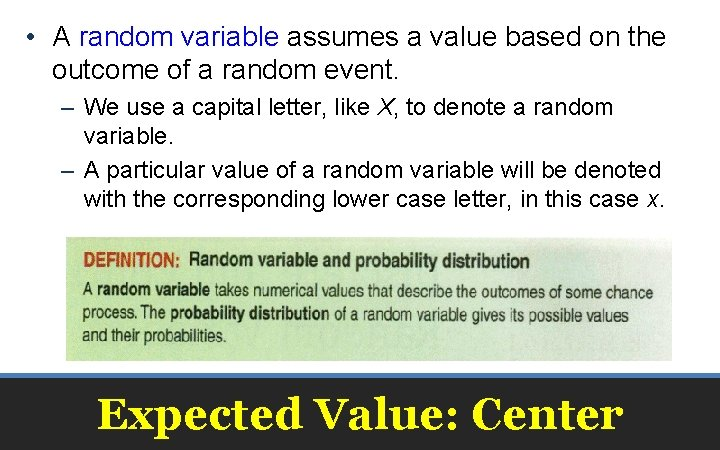• A random variable assumes a value based on the outcome of a random event. – We use a capital letter, like X, to denote a random variable. – A particular value of a random variable will be denoted with the corresponding lower case letter, in this case x. Expected Value: CenterProbability Distributions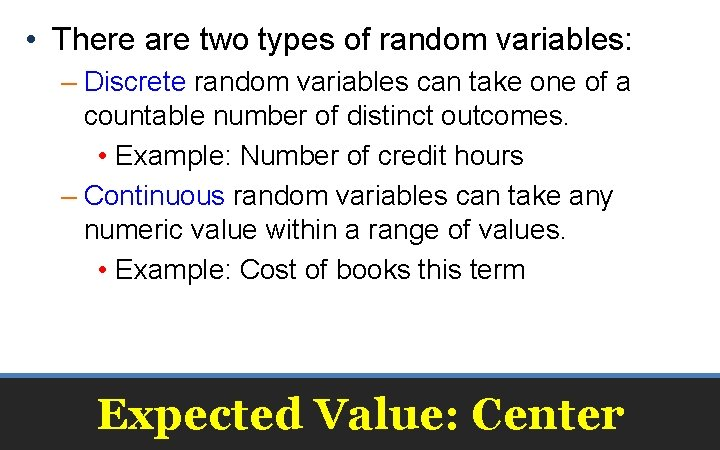• There are two types of random variables: – Discrete random variables can take one of a countable number of distinct outcomes. • Example: Number of credit hours – Continuous random variables can take any numeric value within a range of values. • Example: Cost of books this term Expected Value: Center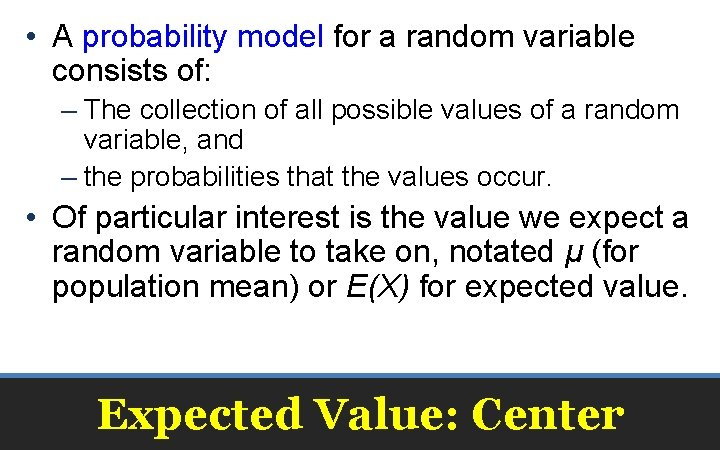• A probability model for a random variable consists of: – The collection of all possible values of a random variable, and – the probabilities that the values occur. • Of particular interest is the value we expect a random variable to take on, notated μ (for population mean) or E(X) for expected value. Expected Value: Center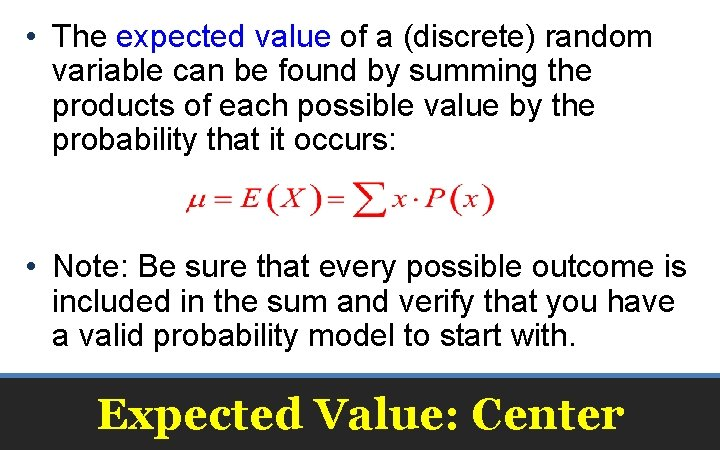• The expected value of a (discrete) random variable can be found by summing the products of each possible value by the probability that it occurs: • Note: Be sure that every possible outcome is included in the sum and verify that you have a valid probability model to start with. Expected Value: Center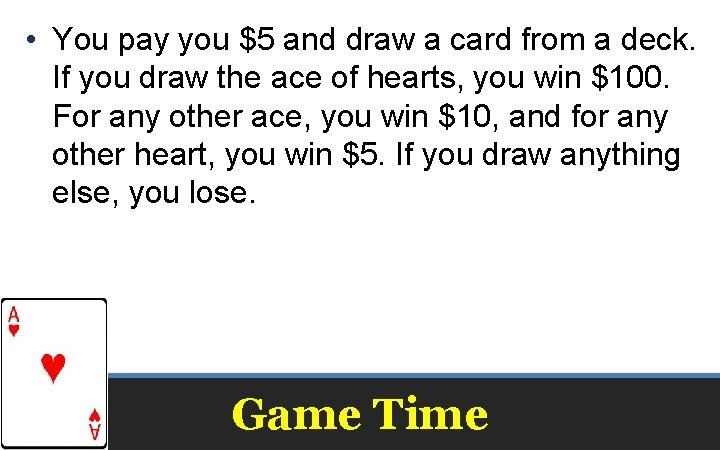• You pay you \$5 and draw a card from a deck. If you draw the ace of hearts, you win \$100. For any other ace, you win \$10, and for any other heart, you win \$5. If you draw anything else, you lose. Game Time• You pay you \$5 and draw a card from a deck. If you draw the ace of hearts, you win \$100. For any other ace, you win \$10, and for any other heart, you win \$5. If you draw anything else, you lose. • Would you play for a top prize of \$200? Game Time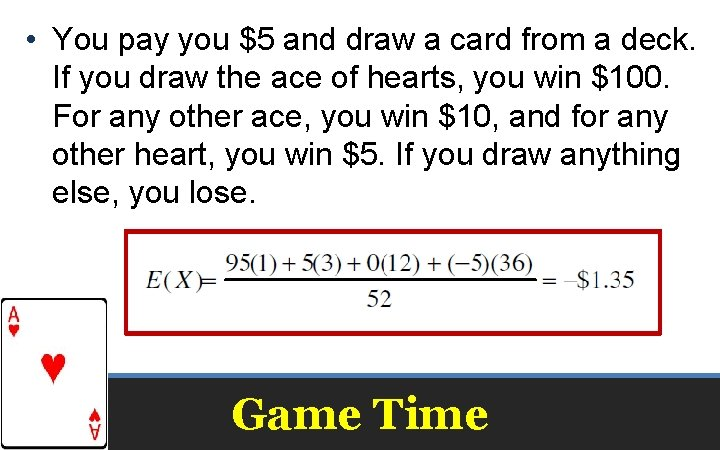• You pay you \$5 and draw a card from a deck. If you draw the ace of hearts, you win \$100. For any other ace, you win \$10, and for any other heart, you win \$5. If you draw anything else, you lose. Game TimeFind SD on your Calculator Game TimeWhy is the SD so high? What would make it even higher? Game Time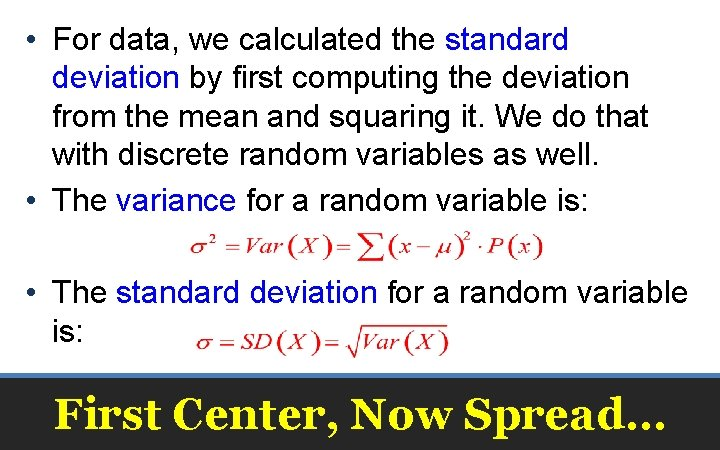• For data, we calculated the standard deviation by first computing the deviation from the mean and squaring it. We do that with discrete random variables as well. • The variance for a random variable is: • The standard deviation for a random variable is: First Center, Now Spread…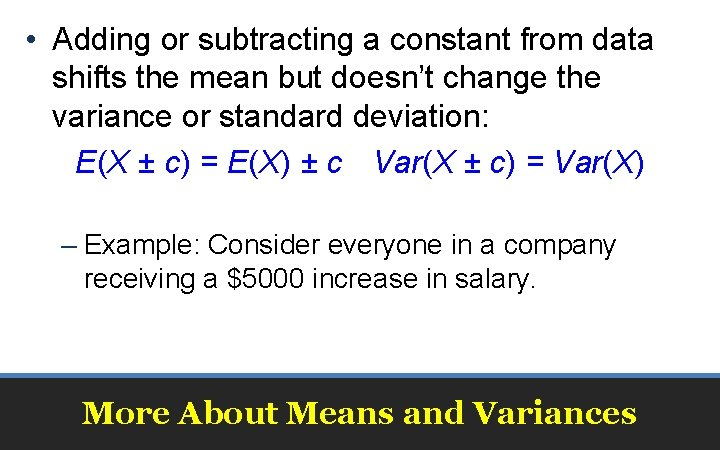• Adding or subtracting a constant from data shifts the mean but doesn’t change the variance or standard deviation: E(X ± c) = E(X) ± c Var(X ± c) = Var(X) – Example: Consider everyone in a company receiving a \$5000 increase in salary. More About Means and Variances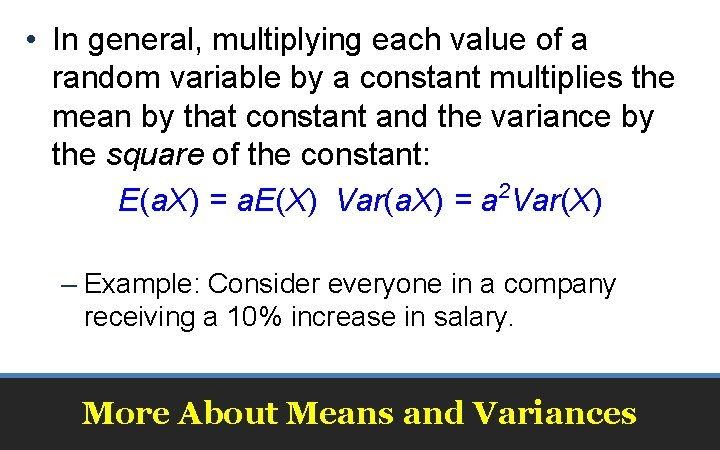• In general, multiplying each value of a random variable by a constant multiplies the mean by that constant and the variance by the square of the constant: E(a. X) = a. E(X) Var(a. X) = a 2 Var(X) – Example: Consider everyone in a company receiving a 10% increase in salary. More About Means and Variances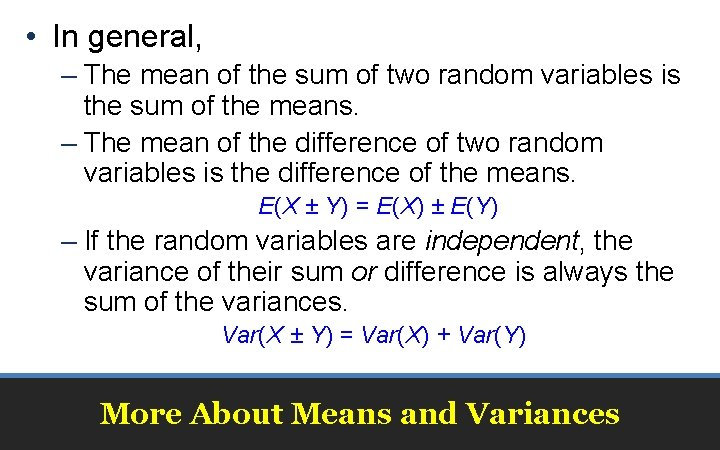• In general, – The mean of the sum of two random variables is the sum of the means. – The mean of the difference of two random variables is the difference of the means. E(X ± Y) = E(X) ± E(Y) – If the random variables are independent, the variance of their sum or difference is always the sum of the variances. Var(X ± Y) = Var(X) + Var(Y) More About Means and Variances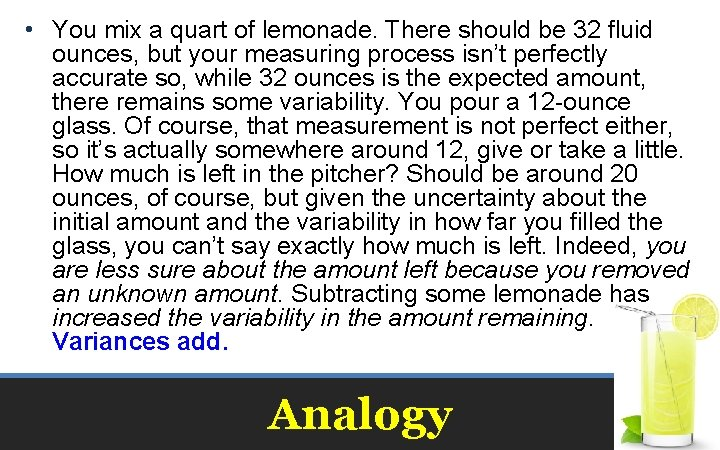• You mix a quart of lemonade. There should be 32 fluid ounces, but your measuring process isn’t perfectly accurate so, while 32 ounces is the expected amount, there remains some variability. You pour a 12 -ounce glass. Of course, that measurement is not perfect either, so it’s actually somewhere around 12, give or take a little. How much is left in the pitcher? Should be around 20 ounces, of course, but given the uncertainty about the initial amount and the variability in how far you filled the glass, you can’t say exactly how much is left. Indeed, you are less sure about the amount left because you removed an unknown amount. Subtracting some lemonade has increased the variability in the amount remaining. Variances add. Analogy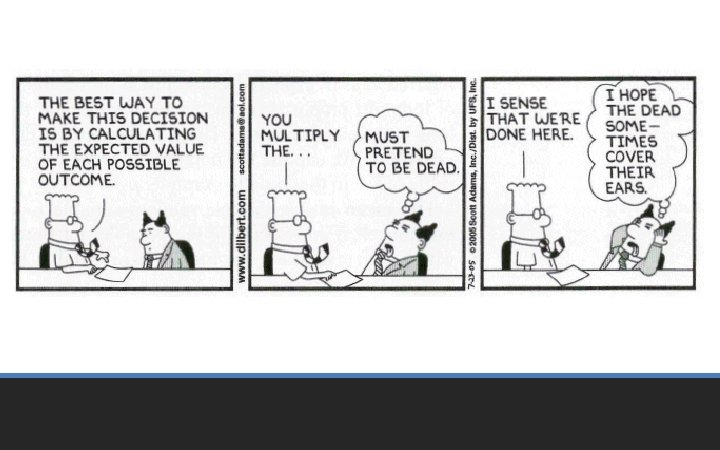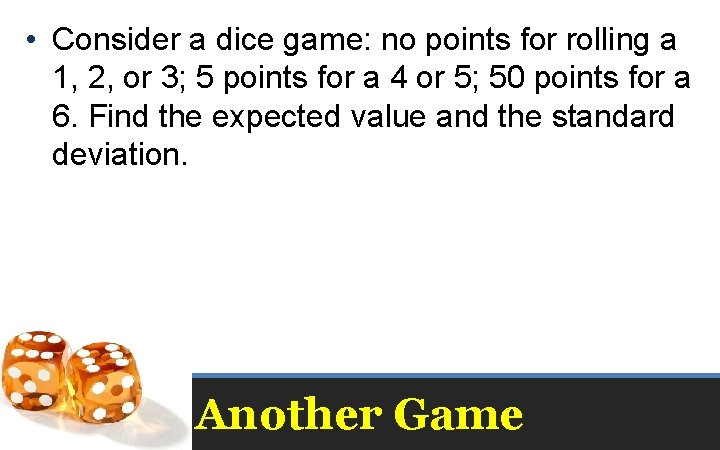• Consider a dice game: no points for rolling a 1, 2, or 3; 5 points for a 4 or 5; 50 points for a 6. Find the expected value and the standard deviation. Another Game• Consider a dice game: no points for rolling a 1, 2, or 3; 5 points for a 4 or 5; 50 points for a 6. Find the expected value and the standard deviation. Another GameI expect to win 10 points per roll in the long run. The standard deviation is 18. 03 points, suggesting a highly variable game. Another Game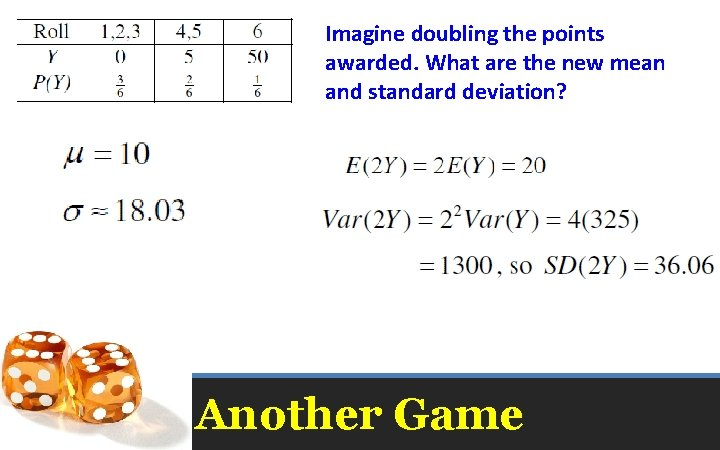Imagine doubling the points awarded. What are the new mean and standard deviation? Another Game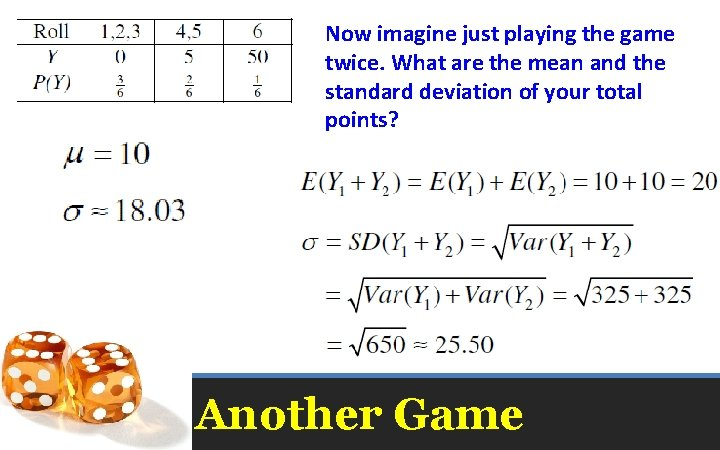Now imagine just playing the game twice. What are the mean and the standard deviation of your total points? Another Game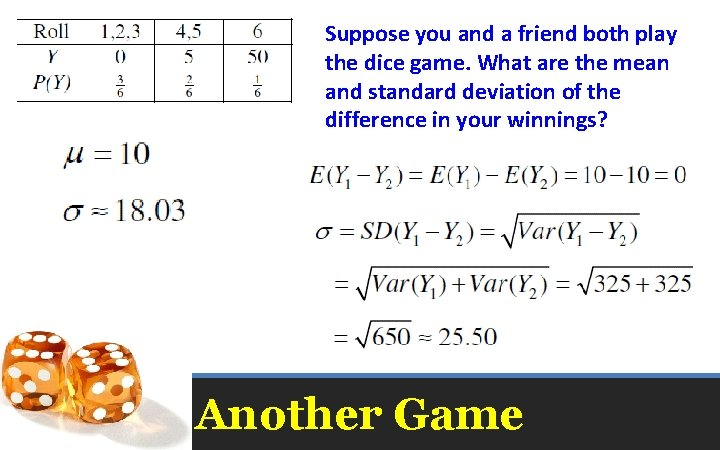Suppose you and a friend both play the dice game. What are the mean and standard deviation of the difference in your winnings? Another Game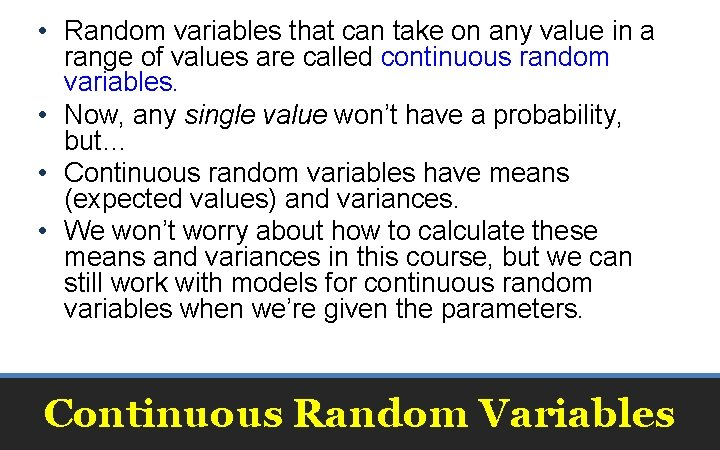• Random variables that can take on any value in a range of values are called continuous random variables. • Now, any single value won’t have a probability, but… • Continuous random variables have means (expected values) and variances. • We won’t worry about how to calculate these means and variances in this course, but we can still work with models for continuous random variables when we’re given the parameters. Continuous Random Variables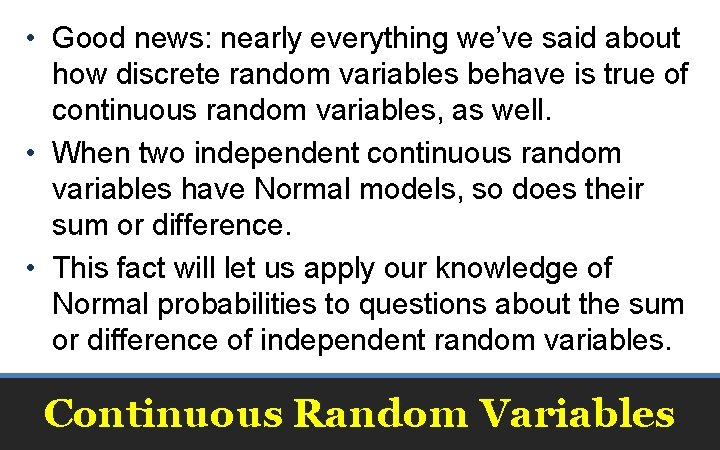• Good news: nearly everything we’ve said about how discrete random variables behave is true of continuous random variables, as well. • When two independent continuous random variables have Normal models, so does their sum or difference. • This fact will let us apply our knowledge of Normal probabilities to questions about the sum or difference of independent random variables. Continuous Random Variables• Probability models are still just models. – Models can be useful, but they are not reality. – Question probabilities as you would data, and think about the assumptions behind your models. • If the model is wrong, so is everything else. What Can Go Wrong?• Don’t assume everything’s Normal. – You must Think about whether the Normality Assumption is justified. • Watch out for variables that aren’t independent: – You can add expected values for any two random variables, but – you can only add variances of independent random variables. What Can Go Wrong?• Don’t forget: Variances of independent random variables add. Standard deviations don’t. • Don’t forget: Variances of independent random variables add, even when you’re looking at the difference between them. • Don’t write independent instances of a random variable with notation that looks like they are the same variables. What Can Go Wrong?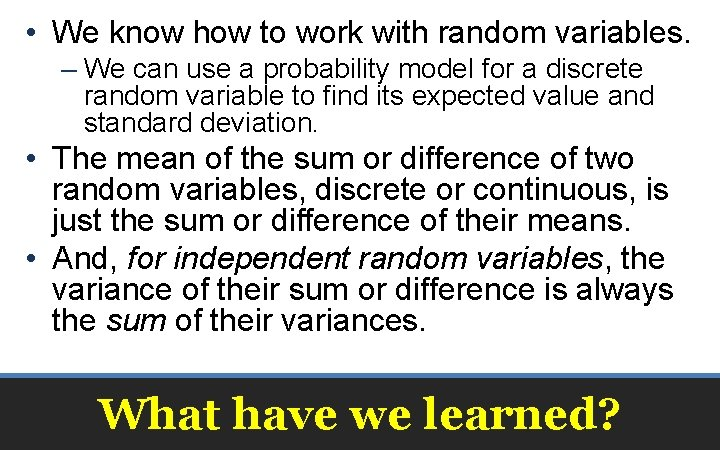• We know how to work with random variables. – We can use a probability model for a discrete random variable to find its expected value and standard deviation. • The mean of the sum or difference of two random variables, discrete or continuous, is just the sum or difference of their means. • And, for independent random variables, the variance of their sum or difference is always the sum of their variances. What have we learned?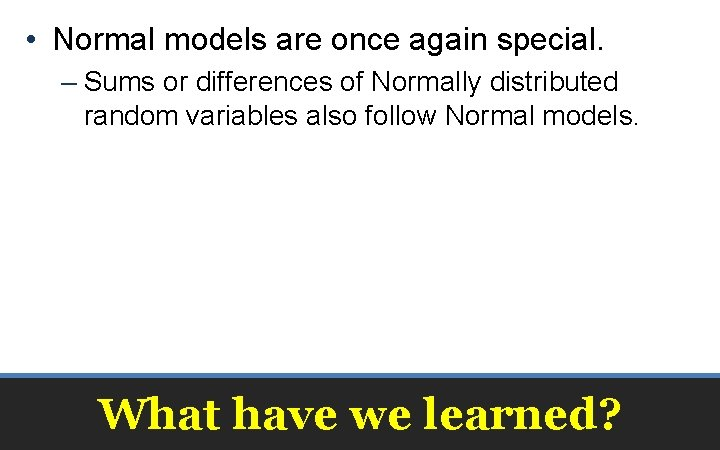• Normal models are once again special. – Sums or differences of Normally distributed random variables also follow Normal models. What have we learned?• Pages 381 – 385; • 2, 3, 5, 7, 10, 11, 13, 16, 20, 22, 24, 25, 32, 38, 40 Homework